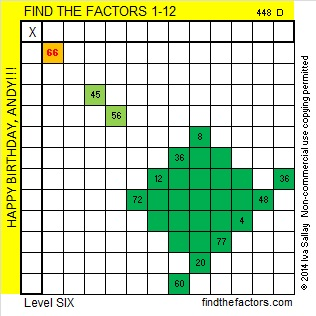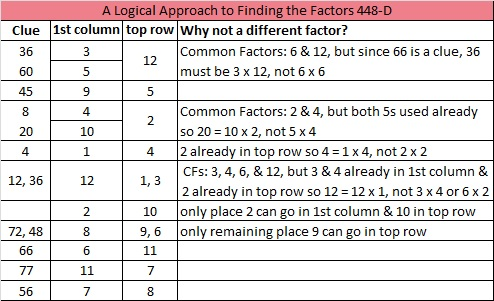# 309 and a Birthday Cake

• 309 is a composite number.
• Prime factorization: 309 = 3 x 103
• The exponents in the prime factorization are 1 and 1. Adding one to each and multiplying we get (1 + 1)(1 + 1) = 2 x 2 = 4. Therefore 309 has exactly 4 factors.
• Factors of 309: 1, 3, 103, 309
• Factor pairs: 309 = 1 x 309 or 3 x 103
• 309 has no square factors that allow its square root to be simplified. √309 ≈ 17.578Happy birthday to my brother, Andy. Enjoy this level 6 cake! Love, IvaPrint the puzzles or type the factors on this excel file: 12 Factors 2014-12-01